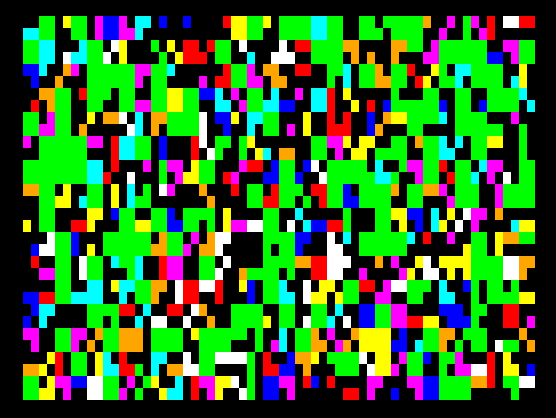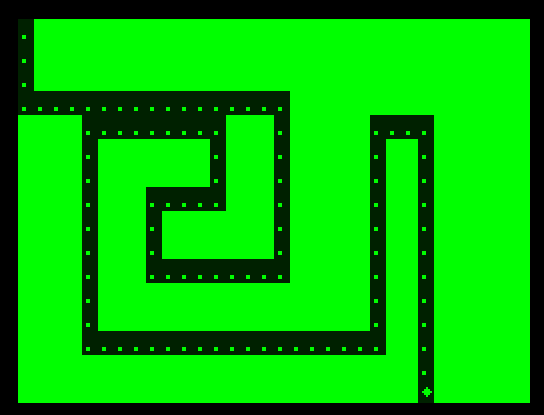# Snippets of Basic Code

These originally appeared on my old Dragon blog - some contributions are attributed to the username Zephyr - you know who you are and thanks again!

## Putting Some Words On The Screen

The easiest way is to get something on the Dragon's text screen is the PRINT command:
```10 PRINT "DRAGON 32 FOREVER"
```
You can also add a margin
```10 PRINT TAB(5) + "DRAGON 32 FOREVER"
```
For most control, use the PRINT @ command
```10 PRINT @200, "DRAGON 32 FOREVER"
```
The number after the @ sign is the position on screen. Top left is 0 and the last character on the screen is 511. Try printing your name around the screen.

## Graphics - Colourful Big Blocks

The Dragon 32 text screen is the most colourful of the machine's display modes. It provides a grid of 32 columns and 16 rows. Text and blocks can be printed on this screen in the same manner - the graphical blocks are just members of the character set.This little snippet gives a confetti display:
```10 CLS
20 G=RND(512)
30 POKE 1023+G,127+RND(128)
40 GOTO 20
```

## Keyboard InputThe following program shows reading the keyboard using a simple method. Each key press is discrete and you have to continually repress the key to move the asterisk character around.
```10 CLS
20 X=0:Y=0
30 POKE 1024+((Y*32)+X),ASC("*")
40 A\$ = INKEY\$
50 IF A\$ = CHR\$(10) THEN GOSUB1000:Y = Y + 1
60 IF A\$ = CHR\$(94) THEN GOSUB1000:Y = Y - 1
70 IF A\$ = CHR\$(9) THEN GOSUB1000:X = X + 1
80 IF A\$ = CHR\$(8) THEN GOSUB1000:X = X - 1
110 IF Y>15 THEN Y=15
130 IF X>31 THEN X=31
200 GOTO 30
1000 REM HIDE IT!
1010 POKE 1024+((Y*32)+X),ASC(".")
1020 RETURN
```
More useful in some situations is to allow the user to press and hold the button for continuous movement. To do this we rely on some PEEK commands which read the keyboard activity straight from the Dragons memory.
```10 CLS
20 X=0:Y=0
30 POKE 1024+((Y*32)+X),ASC("*")
40 PE = PEEK(135) : PR = PEEK(337) : IF PR = 255 THEN PE = 0
50 IF PE = 10 THEN GOSUB1000:Y = Y + 1
60 IF PE = 94 THEN GOSUB1000:Y = Y - 1
70 IF PE = 9 THEN GOSUB1000:X = X + 1
80 IF PE = 8 THEN GOSUB1000:X = X - 1
110 IF Y>15 THEN Y=15
130 IF X>31 THEN X=31
200 GOTO 30
1000 REM HIDE IT!
1010 POKE 1024+((Y*32)+X),ASC(".")
1020 RETURN
```